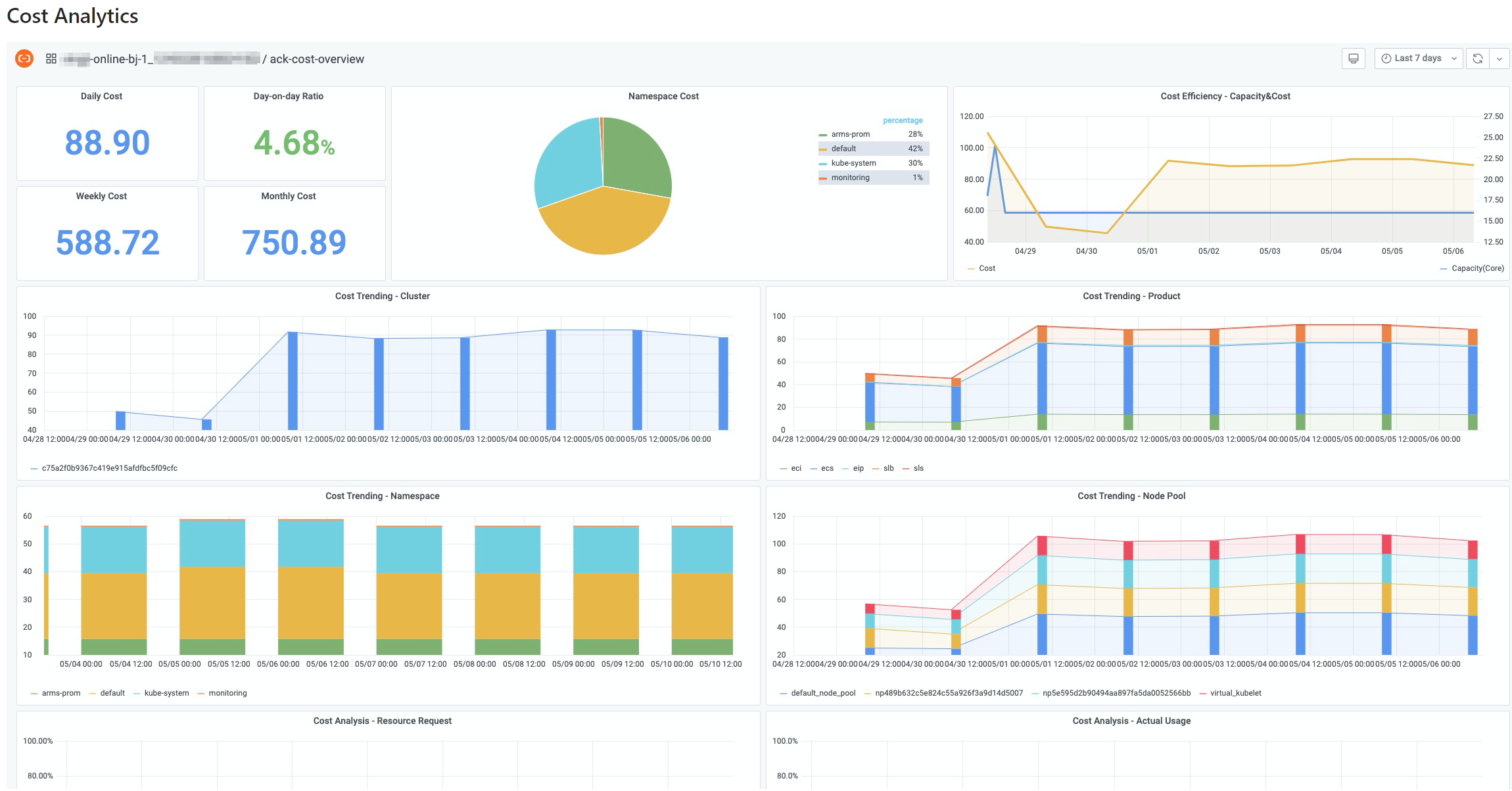This topic introduces the cost analysis feature and describes how to configure cost analysis for a Container Service for Kubernetes (ACK) cluster.

## View cost analysis results

1. Log on to the ACK console and click Clusters in the left-side navigation pane.
2. On the Clusters page, click the name of a cluster and choose Operations > Cost Analysis in the left-side navigation pane.
3. On the Cost Analysis page, click the Cluster tab to view the cluster dashboard that displays the cost analysis results.
Note
• After cost analysis is enabled, bills are displayed at 08:00:00 (UTC+8) on the next day.
• You can click the drop-down list in the upper-right corner of the Cluster tab to change the time range.## Cluster cost analysisThe following table describes the features of the cluster dashboard.

FeatureNumberDescription
Display the cost trend to help you identify resource waste in the clusterThis feature displays information about the cost of a cluster. The Daily Cost metric displays the cost of the previous day. The Weekly Cost metric displays the cost of the previous week. The Monthly Cost metric displays the cost of the previous month. The Day-on-day Ratio metric displays the growth rate of the cost of the previous day.
• If the growth rate is in green, it indicates that the cost of the previous day is reduced compared with the cost of the day before the previous day.
• If the growth rate is in red, it indicates that the cost of the previous day is increased compared with the cost of the day before the previous day.
The yellow curve indicates the resource consumption of the cluster. The blue curve indicates the actual resource capacity of the cluster. In most cases, the two curves are correlated with each other.

If the two curves represent different trends, it indicates changes to the average cost of individual CPU cores. In this case, you can check whether resources of higher prices are consumed.

The cost trend within a time period.
Display the costs of different namespaces

A cluster may contain nodes of different specifications and billing methods. When you allocate the cost based on namespaces, you must consider the price differences of nodes that host pods in different namespaces, not only the resource consumption of different namespaces.

The cost analysis feature converts the real-time cost of each node and provides the following formula to calculate the cost of a namespace:
``Σ (Pod resource request/Node capacity) × Node unit price``

The formula can help you precisely calculate the cost of a namespace. However, due to the deferred billing policy applied when discounts, vouchers, or the subscription billing method is used, the cost calculated based on this formula may differ from the amount on the bill. To calculate the actual cost of a namespace, you can multiply the amount on the cluster bill by the cost ratio of the namespace.

Display the cost trends of cloud resourcesA cluster may use multiple cloud resources. The costs of cloud resources vary based on billing rules and how the cloud resources are used by the cluster. This feature displays the cost trends and contributions of different cloud resources to help you reduce the cost of the cluster.

## FAQ

Answer: Check whether the RAM role is granted the required permissions. For more information, see Step 5 in the Enable cost analysis topic. Cost analysis is based on monitoring data and cost data. The collection of monitoring data starts within 3 minutes after you enable cost analysis. Cost data is displayed at 08:00:00 (UTC+8) on the next day.
Answer: The cost of a namespace is calculated based on cost estimation and is not directly related to the actual bills. Therefore, the cost estimation is based on the list prices. However, the actual cost of a cluster can be reduced by vouchers, discounts, and cost saving plans. As a result, the total estimated cost of all namespaces may not equal the actual cost of the cluster. To obtain the actual cost of a namespace, you can multiply the actual cost of the cluster by the cost ratio of the namespace.
Answer: Check whether the RAM role is granted the required permissions. Make sure that the ecs:DescribeDisks permission is included in the permission policy. For more information, see Step 5 in the Enable cost analysis topic.
Answer: Cost analysis only collects information about cloud services that are exclusive to your cluster. Information about cloud services that are shared by multiple clusters is not collected.